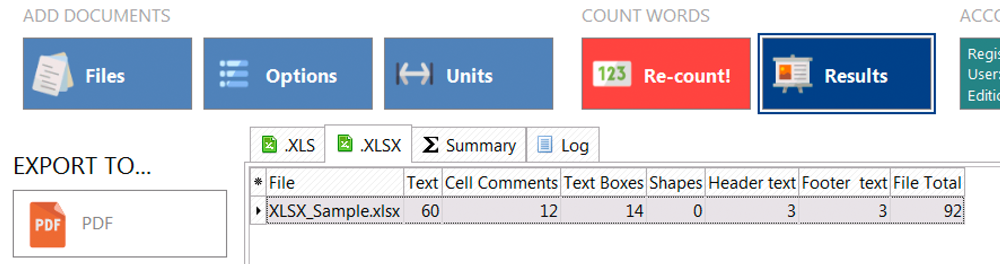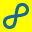W O R D C O U N T ? A N Y C O U N T !Usually, professionals use Microsoft Excel to record expenses, income, plan budgets, and present their fiscal outcomes, but translators and editors often face the need to count text. Would it surprise you to learn that to сount text in Excel is not an easy task? But since you are reading this post, you have already face Excel word count challenges.

The software doesn’t have a magic button to click on and view all character and word count statistics. You need particular formulas, just like for any other calculations in Excel. This post will show you two ways to count words in Excel and offer you an alternative way to count text in the document body, cell comments, text boxes, shapes, header, and footer.

### The formula for counting words in a cell

You can find a range of different formulas for word count in a cell. We tried the most common, and here are the results.

Generic formula: =LEN(A1)-LEN(SUBSTITUTE(A1,” “,””))+1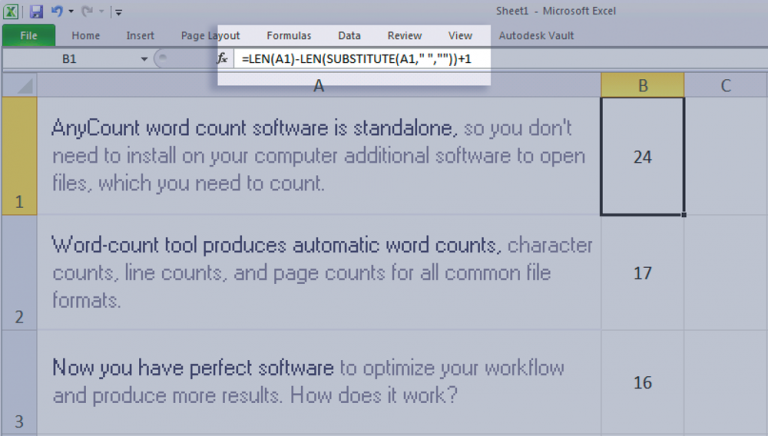Formula based on the LEN and SUBSTITUTE functions:

=LEN(TRIM(A1))-LEN(SUBSTITUTE(A1,” “,””))+1### Protect your text count results from false positives

One of the drawbacks of these formulas is that even in an empty cell, they will show one word instead of zero. Therefore, if you have blank cells, you need the formula to take this condition into account. To protect your results from this problem, use IF and ISBLANK: =IF(ISBLANK(A1),0,LEN(TRIM(A1))-LEN(SUBSTITUTE(A1,” “,””))+1)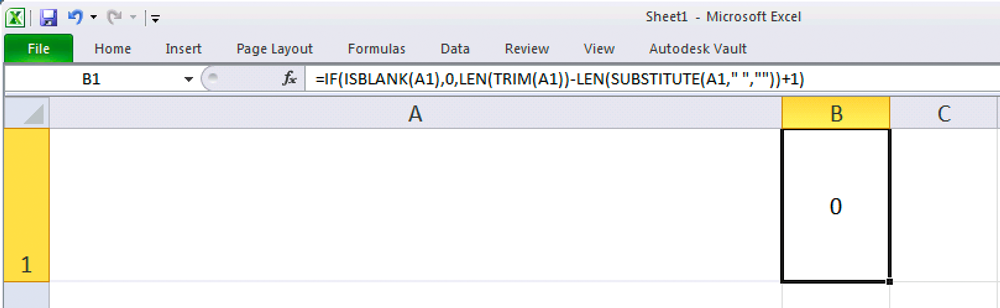### So what's the catch?

Excel doesn’t understand what a word is. It cannot count them. So what do the formulas do? The trick is that formula allows you to calculate the spaces between words plus one to the result. Since there are fewer spaces than words, this usually works. But how accurate is it?

If for some reason, your word count is not equal to spaces plus 1, you will get false results. So this method is somewhat unreliable.

### Count words in a range of cells

Now let’s move to the next level. Here you will need to count the words already in a range of cells instead of one cell. Good news! You can use the same formula (with a slight change) that we used above. Here it is: =SUMPRODUCT(LEN(A1:A3)-LEN(SUBSTITUTE(A1:A3,” “,””))+1)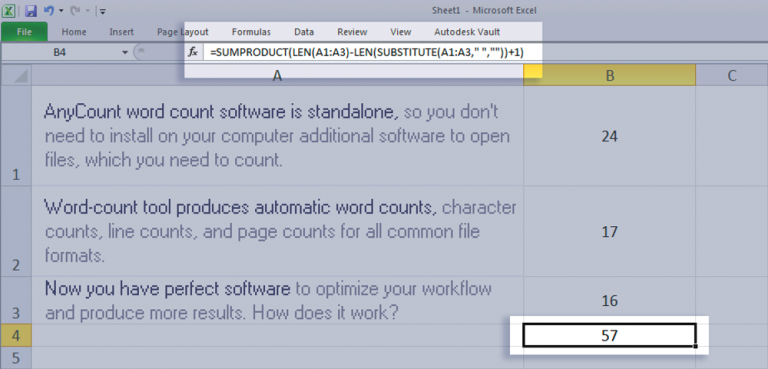If you have already counted words for each cell, you can always use the SUM function and sum up all values.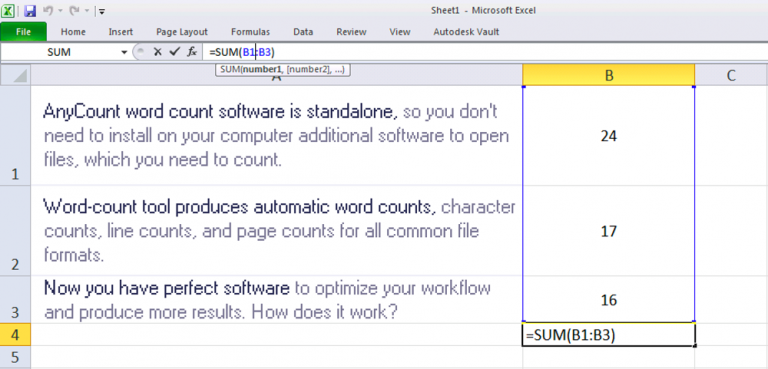### Word count tool to count text in Excel files

If your income depends on accurate word counting, plus you don’t want to waste your time using formulas, it is better to use the word count tool to count words quickly and accurately. Download AnyCount for free, drag the Excel files into the Anycount window, and in a few seconds, you will get word count statistics.

You can view text count and more detailed data about cell comments, text boxes, word count in shapes, header, and footer text.